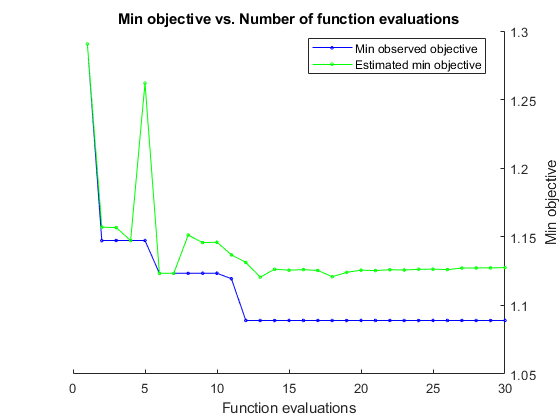Main Content

# Tune Random Forest Using Quantile Error and Bayesian Optimization

This example shows how to implement Bayesian optimization to tune the hyperparameters of a random forest of regression trees using quantile error. Tuning a model using quantile error, rather than mean squared error, is appropriate if you plan to use the model to predict conditional quantiles rather than conditional means.

### Load and Preprocess Data

Load the `carsmall` data set. Consider a model that predicts the median fuel economy of a car given its acceleration, number of cylinders, engine displacement, horsepower, manufacturer, model year, and weight. Consider `Cylinders`, `Mfg`, and `Model_Year` as categorical variables.

```load carsmall Cylinders = categorical(Cylinders); Mfg = categorical(cellstr(Mfg)); Model_Year = categorical(Model_Year); X = table(Acceleration,Cylinders,Displacement,Horsepower,Mfg,... Model_Year,Weight,MPG); rng('default'); % For reproducibility ```

### Specify Tuning Parameters

Consider tuning:

• The complexity (depth) of the trees in the forest. Deep trees tend to over-fit, but shallow trees tend to underfit. Therefore, specify that the minimum number of observations per leaf be at most 20.

• When growing the trees, the number of predictors to sample at each node. Specify sampling from 1 through all of the predictors.

`bayesopt`, the function that implements Bayesian optimization, requires you to pass these specifications as `optimizableVariable` objects.

```maxMinLS = 20; minLS = optimizableVariable('minLS',[1,maxMinLS],'Type','integer'); numPTS = optimizableVariable('numPTS',[1,size(X,2)-1],'Type','integer'); hyperparametersRF = [minLS; numPTS]; ```

`hyperparametersRF` is a 2-by-1 array of `OptimizableVariable` objects.

You should also consider tuning the number of trees in the ensemble. `bayesopt` tends to choose random forests containing many trees because ensembles with more learners are more accurate. If available computation resources is a consideration, and you prefer ensembles with as fewer trees, then consider tuning the number of trees separately from the other parameters or penalizing models containing many learners.

### Define Objective Function

Define an objective function for the Bayesian optimization algorithm to optimize. The function should:

• Accept the parameters to tune as an input.

• Train a random forest using `TreeBagger`. In the `TreeBagger` call, specify the parameters to tune and specify returning the out-of-bag indices.

• Estimate the out-of-bag quantile error based on the median.

• Return the out-of-bag quantile error.

```function oobErr = oobErrRF(params,X) %oobErrRF Trains random forest and estimates out-of-bag quantile error % oobErr trains a random forest of 300 regression trees using the % predictor data in X and the parameter specification in params, and then % returns the out-of-bag quantile error based on the median. X is a table % and params is an array of OptimizableVariable objects corresponding to % the minimum leaf size and number of predictors to sample at each node. randomForest = TreeBagger(300,X,'MPG','Method','regression',... 'OOBPrediction','on','MinLeafSize',params.minLS,... 'NumPredictorstoSample',params.numPTS); oobErr = oobQuantileError(randomForest); end ```

### Minimize Objective Using Bayesian Optimization

Find the model achieving the minimal, penalized, out-of-bag quantile error with respect to tree complexity and number of predictors to sample at each node using Bayesian optimization. Specify the expected improvement plus function as the acquisition function and suppress printing the optimization information.

```results = bayesopt(@(params)oobErrRF(params,X),hyperparametersRF,... 'AcquisitionFunctionName','expected-improvement-plus','Verbose',0); ````results` is a `BayesianOptimization` object containing, among other things, the minimum of the objective function and the optimized hyperparameter values.

Display the observed minimum of the objective function and the optimized hyperparameter values.

```bestOOBErr = results.MinObjective bestHyperparameters = results.XAtMinObjective ```
```bestOOBErr = 1.0890 bestHyperparameters = 1×2 table minLS numPTS _____ ______ 7 7 ```

### Train Model Using Optimized Hyperparameters

Train a random forest using the entire data set and the optimized hyperparameter values.

```Mdl = TreeBagger(300,X,'MPG','Method','regression',... 'MinLeafSize',bestHyperparameters.minLS,... 'NumPredictorstoSample',bestHyperparameters.numPTS); ```

`Mdl` is `TreeBagger` object optimized for median prediction. You can predict the median fuel economy given predictor data by passing `Mdl` and the new data to `quantilePredict`.

Download ebook